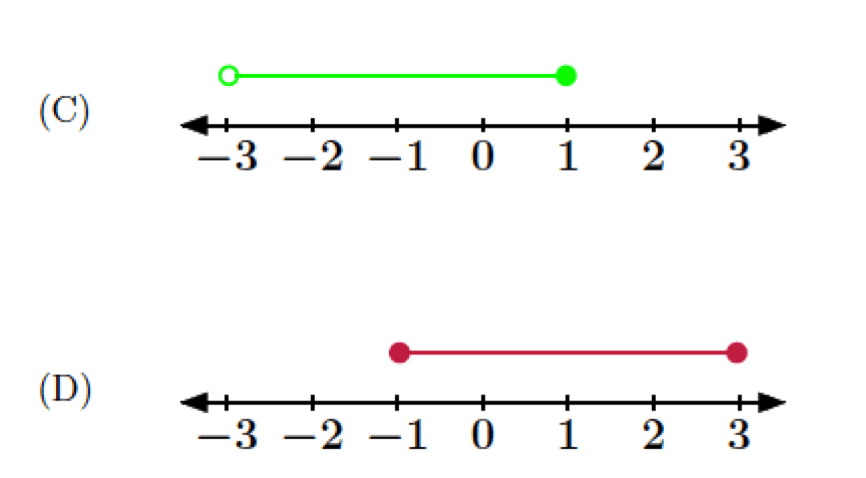# RATL 3 | Lesson 2 | Explore (Solution Sets)In this lesson you will learn to solve rational inequalities. One we get a solution set, there are several different ways to express a solution set. An example is the following written in basic style:

$$-7\leq{x}<4$$.

This same solution set can be represented in:

a)  set builder notation as $$\{x|-7\leq{x}<4\}$$.

b) interval notation as $$[-7, 4)$$.

From the number lines below, write the solution set in basic notation,  set builder notation and interval notation.  Put this information in the chart on the bottom of this page.Problem Basic Style Set Builder Interval
a
b
c
d